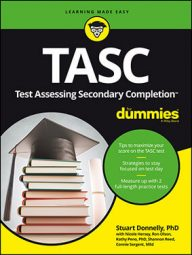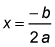##### TASC For DummiesIf you're given the standard form of a quadratic equation on the TASC Math exam, the equation provides valuable information when you're asked to graph it.

A quadratic function is a polynomial in which the highest degree is two. The shape of the graph representing the quadratic function is called a parabola, which is a U shape.

You can create a table, select values for the independent variable (x), and substitute in and solve for the values of the dependent variable (y). Similar to how it takes two points to construct a line, you need a minimum of three points to make a parabola.

The standard form of a quadratic function is y = ax2 + bx + c.

1. Determine the axis of symmetry(this is also the x-coordinate of the vertex).
2. Substitute the value obtained in Step 1 back into the original formula to determine the y-coordinate of the vertex.
3. Pick two points that are equidistant from the x-coordinate of the vertex.
4. Substitute these values into the original formula (these resulting y-coordinates should be the same if the x-coordinates are the same distance away). Try to pick when x = 0 as one of the values because doing so simplifies the algebra.
5. Plot the three points (vertex and two points from Step 4) on the coordinate plane.
6. Connect the points you plotted in a smooth curve (careful, you don't want to make the graph pointy or V-shaped).
Here's an example:

y = 2x2 – 4x + 3

a = 2 b = –4 c = 3

y coordinate:

y = 2(1)2 – 4(1) + 3

y = 2(1) – 4 + 3

y = 1

vertex: (1, 1)

Because 0 is one away from 1, you pick 2 as the other point. To obtain the y-values, substitute 0 and 2 back into y = 2x2 – 4x + 3 and solve.

Stuart Donnelly, PhD, was awarded a PhD in mathematics from Oxford University. He has prepared students for the TASC test and GED Test for the past two decades.

Stuart Donnelly, PhD, earned his doctorate in mathe-matics from Oxford University at the age of 25. Since then, he has established successful tutoring services in both Hong Kong and the United States and is considered by leading educators to be one of the most experienced and qualified private tutors in the country.

Stuart Donnelly, PhD, was awarded a PhD in mathematics from Oxford University. He has prepared students for the TASC test and GED Test for the past two decades.

Stuart Donnelly, PhD, was awarded a PhD in mathematics from Oxford University. He has prepared students for the TASC test and GED Test for the past two decades.

Sandra Luna McCune, PhD, is professor emeritus and a former Regents professor at Stephen F. Austin State University. She's now a full-time author. Shannon Reed, MA, MFA, is a visiting lecturer at the University of Pittsburgh, where she teaches composition, creative writing, and business writing.

Stuart Donnelly, PhD, was awarded a PhD in mathematics from Oxford University. He has prepared students for the TASC test and GED Test for the past two decades.